Courses

# Chapter 3 Corona - Notes, Power System, Electrical Engineering Electrical Engineering (EE) Notes | EduRev

## Electrical Engineering (EE) : Chapter 3 Corona - Notes, Power System, Electrical Engineering Electrical Engineering (EE) Notes | EduRev

The document Chapter 3 Corona - Notes, Power System, Electrical Engineering Electrical Engineering (EE) Notes | EduRev is a part of the Electrical Engineering (EE) Course Electrical Engineering SSC JE (Technical).
All you need of Electrical Engineering (EE) at this link: Electrical Engineering (EE)

Corona

INTRODUCTION

Corona phenomenon is defined as a self-sustained electric discharge in which the field intensified ionization is localized only over a portion of the distance between electrodes. 

• It is a phenomena of ionization of a air surrounding the power conductor. 
• It is characterized by a point glow, a crackling and conversion of atmospheric oxygen to ozone.

CRITICAL DISRUPTIVE VOLTAGE

The voltage at which complete disruption of dielectricoccurs is called critical disruptive voltage.

Remember: 

• This voltage corresponds to the gradient at the surface equal to the breakdown strength of air. 
• Corona begins when the peak value of critical field intensity equals 30 kV/cm in case of smooth conductors of large diameter in air at NTP (i.e. 25°C and 76 cm of Hg).
• Relative density of air at temperature q°C and barometric pressure of p cm of Hg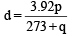•  It depends upon the density of air and the surface of the conductor.

Remember: 

• The critical intensity is proportional to density of air. 
• Roughness of conductor surface causes a distortion in electric field and gives rise to local regions of high potential gradient resulting in a lowering of the critical intensity. 
• RMS value of critical intensity after taking the effect of temperature, pressure and surface condition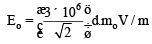Where mo is the roughness factor of conductor surface.

Note: 

• Roughness factor of conductor surface is 1 for smooth conductor and 0.93 to 0.98 for rough surface exposed to atmosphere. 
• Critical disruptive voltage for a single phase line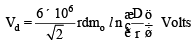Where r is the radius of conductors spaced by distance D. 

• For a 3-f line with equivalent spacing Deq, the phase value of critical disruptive voltage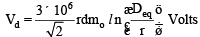VISUAL CRITICAL VOLTAGE

Visual corona does not occurs when the electric intensity becomes equal to ciritical value Eo but startsat a higher value of intensity Ev, because the charged ions in the air must receive some finite energy to cause further ionization by collisions. 

• Visual critical intensity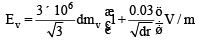Where mv is the irregularity factor of the conductor surface. 

• Visual critical voltage For 1-f line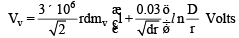For 3-f line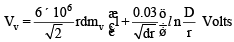Note: 

• The value of irregularity factor is 0.72 for local corona and 0.82 for decided corona on standard conductors.

CORONA LOSS

It is the amount of electric power lost in high voltage lines due to the rediation of energy by corona discharge.

Remmber: 

• The cor ona los s d oes not have any appreciable effect on voltage regulation but affects the efficiency of the transmission line.

Peek’s Formula 

• Corona Loss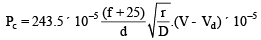Where Pc = Corona loss in kW/km/phase
V = Rms value of phase voltage in
kV Vd = RMS value disruptive critical voltage in
kV r = Radius of conductor in metres
D = Spacing (or equivalent spacing) between conductor in metres
f = System frequency

Note: 

• Above formula is valid for fair weather conditions under stormy weather conditions the corona loss is calculated by taking Vd as 80% of fair weather condition.

Peterson’s Formula 

• Corona Loss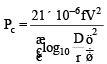Where F is a factor which varies with ratio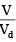Remember:

• Peterson’s formula is used whenis less than 1.8.
• Peek’s formula is validis greater than
1.8.

Factors Affecting Corona Loss

Effect of Frequency

• Corona loss is directly proportional to system frequency

Effect of System Voltage

• Greater the potential difference, greater is the electric field and greater is the corona loss. ¡ When V is large as compared with Vd, corona loss increases at a very fast rate with increase in voltage.

Effect of Conductivity of Air

• The conductivity of air depends on the number of ions per unit volume of air, the size and charge per ion.
• Higher conductivity leads to greater corona loss.

Effect of Density of Air

• Corona loss increases with decrease in density of air.
• The corona loss of a line passing through a hilly area is higher than that of a similar line in plains due to the decreased value of d at high altitudes.

Effect of Rain, Dust, etc.

• Rain and dust increase the corona loss.
• The effect is more pronounced if the conductor diameter is large.

• A higher conductor radius means lesser surface field intensity and, hence, reduced corona loss.

Remember: 

• ACSR conductor lines have lower corona loss than copper conductor lines.
• In bundled conductor lines the effective radius is high and, therefore these lines depict lower corona loss than the lines with one conductor per phase

Effect of Conductor Surface

• Any roughness on the conductor surface causes a field distortion and gives rise to local regions of high potential gradient and higher corona loss as compared to smooth surface.
Offer running on EduRev: Apply code STAYHOME200 to get INR 200 off on our premium plan EduRev Infinity!

65 docs|37 tests

,

,

,

,

,

,

,

,

,

,

,

,

,

,

,

,

,

,

,

,

,

,

,

,

,

,

,

;